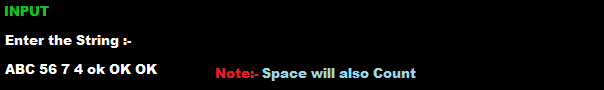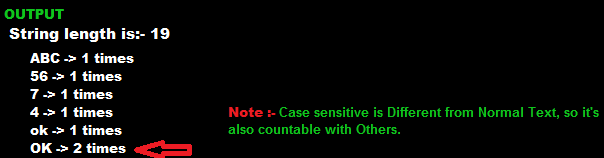# Count Total Number of Words and Character in string in c

Count Number of string Data Multiple time and Number of Character and Word in C Programming String with total inputted Word and Character with space, Count Total Number of Words and Character in string in C

## C Program to Find the Frequency of Characters in a String

#include <stdio.h>

#include <string.h>

void main()
{
int count = 0, c = 0, i, j = 0, k, space = 0;

char str, p, str1, ptr1;

char *ptr;

printf(“Enter the string\n”);
scanf(” %[^\n]s”, str);

printf(“string length is %d\n”, strlen(str));

for (i = 0;i<strlen(str);i++)
{
if ((str[i] == ‘ ‘)||(str[i] == ‘,’ && str[i+1] == ‘ ‘)||(str[i] == ‘.’))
{
space++;
}
}

for (i = 0, j = 0, k = 0;j < strlen(str);j++)
{
if ((str[j] == ‘ ‘)||(str[j] == 44)||(str[j] == 46))
{
p[i][k] = ‘\0’;
i++;
k = 0;
}
else
p[i][k++] = str[j];
}

k = 0;

for (i = 0;i <= space;i++)
{
for (j = 0;j <= space;j++)
{
if (i == j)
{
strcpy(ptr1[k], p[i]);
k++;
count++;

break;
}
else
{
if (strcmp(ptr1[j], p[i]) != 0)
continue;
else
break;
}
}
}

for (i = 0;i < count;i++)
{
for (j = 0;j <= space;j++)
{
if (strcmp(ptr1[i], p[j]) == 0)
c++;
}
printf(“%s -> %d times\n”, ptr1[i], c);
c = 0;
}
}

## Count the total number of words in a stringCount Total Number of Words and Character in string in c

### Count Number of Character and Word in C Programming

This program will count all of String Data including SPACE, with repeated value, for example, if you enter HELLO, with capital latter then it will count it Once. And if you enter simply hello twice in small latter then it will count is like 2 times

### Count a String with total inputted Word and Character in CCount Total Number of Words and Character in string in c

#### Count character with space in C Program

END

C program to draw a square using graphics (clickHere)

Note:- Maybe sometime above code doesn’t get user-friendly output, in codeblock ‘or’ any other EDITOR, All of C & C++ program tested in DOS compiler Turbo C.

## Types of functions in C    Read Here

I hope you will understand better if you study at M.U, in the exam of M.U you know how important this “Count Total Number of Words and Character in string in C. So please don’t forget to give your valuable feedback. Go in the comment section and Give us some feedback about it. Count Total Number of Words and Character in string in C or My website.

Our team check your every Comment per day Because your one feedback helps us in growing Thesmolt

I request you if you have any doubt or question regarding our site, then please don’t hesitate. We always waiting for your Suggestion.

Note:- If you want to Download This article’s in PDF form, then simply go (Top or Bottom of) Right corner and click on the PRINT icon, and it’s saved as a PDF of Count Total Number of Words and Character in string in C